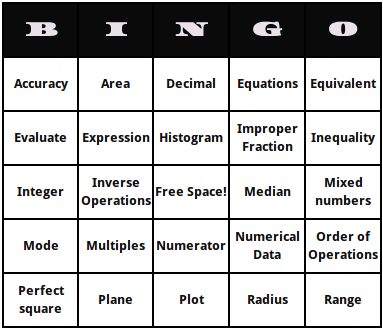B I N G O Free Space!These are fifth grade math terms bingo cards. They consist of math terms that fifth graders should be familiar with like "Equations", "Radius" and advanced math words like "Median".

Generate the fifth grade math terms bingo cards by clicking the "Generate Bingo Cards" button. Add more fifth math terms to the word list or remove any (but must be at least 24 terms to fill the card).

You can also:

Words for 5th Grade Math Terms Bingo Cards

 Accuracy Area Decimal Equations Equivalent Evaluate Expression Histogram Improper Fraction Inequality Integer Inverse Operations Median Mixed numbers Mode Multiples Numerator Numerical Data Order of Operations Perfect square Plane Plot Radius Range Sample Space Stem and leaf Variable Weight

### Features

• Unlimited bingo cards